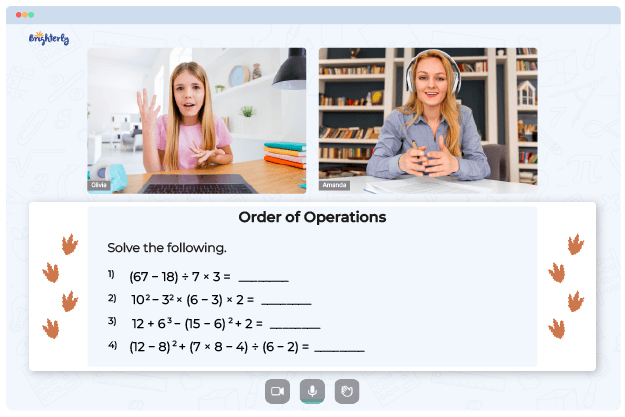# Order Of Operations Worksheets

At the beginning of elementary school, children learn how to do essential addition, subtraction, multiplication, and division of two numbers (1 + 1, 2 x 5, etc.). But what happens when they encounter more complex operations like 14 + 2 – 3 x 4? To ensure kids don’t get stuck, wise teachers introduce them to an order of operations worksheet early on.

## Why to Use the Order of Operations Worksheets from Brighterly?

The order of operations is a collection of principles for choosing which operation to do first. The sequence in which operations are performed illustrates procedural mathematics well. There are a lot of rules kids have to learn, so they might get confused and fail the first time.

Brighterly is an online learning platform whose tutors hold fun and carefully controlled math classes for elementary kids. So, it is no surprise that the tutors understand the need for using order of operations worksheets to reinforce students’ understanding.

Remembering the sequence of operations in math is essential. Worksheets help students understand and use that sequence to solve multi-operational problems. The math procedures used to solve mathematical problems are always performed in the same sequence (i.e., parentheses, exponents, multiplication and division, addition and subtraction).

Math for Kids

Is Your Child Struggling With Math?
1:1 Online Math TutoringEach stage of the PEMDAS mnemonic is progressively introduced in the worksheets. Whether or not parentheses are present, tutors advise students to carry out basic arithmetic operations like adding, subtracting, multiplying, and dividing in the traditional sequence. The creators of the worksheets aim to make your child a pro at identifying the correct sequence of operations for any math question.

Order of operations worksheets with answers allow your child to crosscheck solutions and make corrections. The answer keys ensure a child learns from their mistakes.

Helping your kid with online math lessons for 4th grade. Expert tutors inspiring kids to love math so they can excel at It.

## Why an Order of Operations Worksheet Works

Kids can use order of operations worksheets in PDF. They can move from a basic to advanced order of operations worksheet that includes parentheses [()] and exponents (², ³, ⁴, ⁵, etc.) Worksheets are designed in a way to give students a solid foundation.

Students can only do mathematical parentheses and exponents when they are confident in the sequence of operations. Meanwhile, they can revisit multiplication, division, addition, and subtraction rules to demonstrate how parenthesis influences the order of operations. Afterward, a child can study independently, and if they get questions wrong, they get to retry.### Order Of Operations Worksheets PDF

Order Of Operations Worksheet### Order Of Operations Worksheets PDF

Order Of Operations Worksheets With Answers

Worksheets also open the door to many fun activities kids can do while working on math problems. Using an order of operations worksheet in PDF format, parents can make photocopies so their kids can repeat specific questions from a worksheet.

When children finally perfect all questions, they can use a stopwatch to time themselves and increase their speed. This way, they can work faster and independently when returning to school.

### Order of Operations Worksheets

Need help with Order of Operations?• Does your child need extra help with mastering of order of operations?
• Start studying with an online tutor.

Is your child finding it hard to understand the concept of order of operations? An online tutor could be of help.